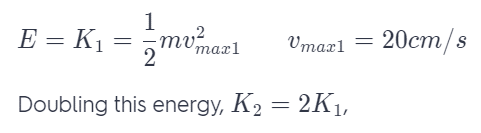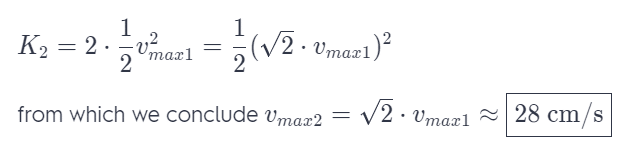# A Block Oscillating On A Spring Has A Maximum Speed Of 20 Cm/S.

We thoroughly check each answer to a question to provide you with the most correct answers. Found a mistake? Let us know about it through the REPORT button at the bottom of the page.

A block oscillating on a spring has a maximum speed of 20 cm/s. What will the block’s maximum speed be if the total energy is doubled? Explain.

## Explanation

The total energy (kinetic+ potential) during motion is constant, equal at any displacement.

At x – =, the equilibrium point, where U = 0, v = vmax = 20cm/s, where all the energy is kinetic energy we have: